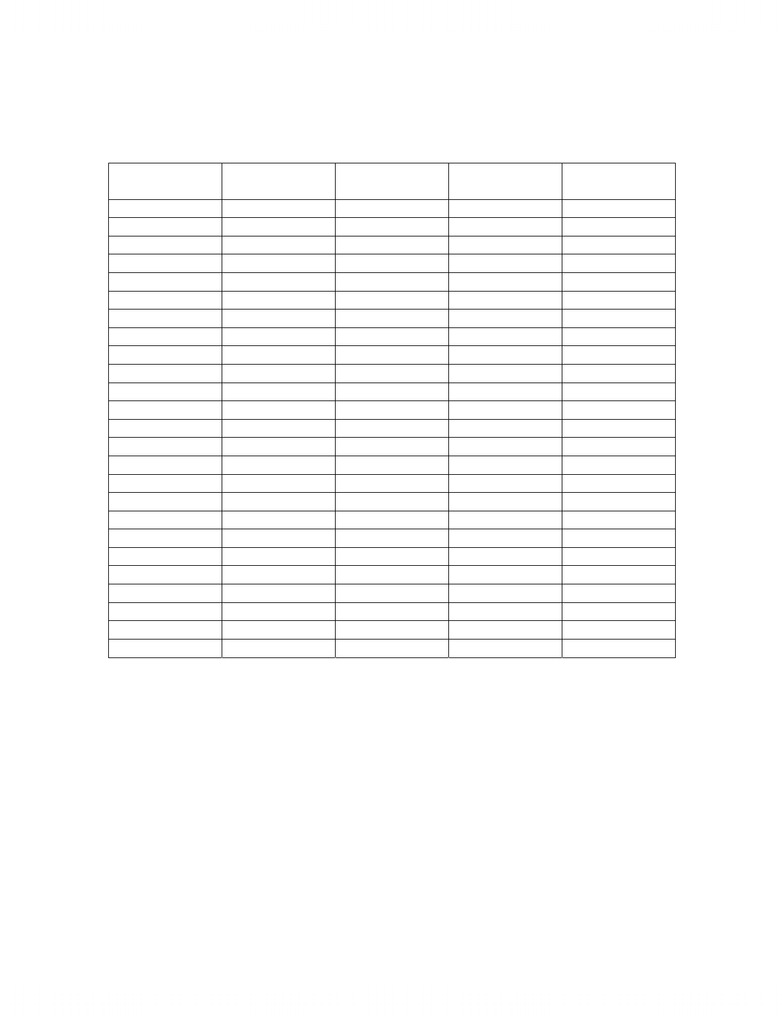# Economics 2150A/B Study Guide - Final Guide: Factor Cost, Pareto Efficiency, Gdp Deflator

146 views6 pages
School
Department1
Economics152b-003
Solutions to Midterm 1, February 2007
Version 222
Version 333 Version 444 Version 555
1. C B A A
2. D C B B
3. B E D and E* B
4. B C C E
5. A A A E
6. B B B E
7. C D and E* B E
8. E C E E
9. C A E E
10. A B E D
11. B B E E
12. D and E* E E B
13. C E E C
14. A E D D
15. B E E B
16. B E B B
17. E E C A
18. E D D B
19. E E B C
20. E B B E
21. E C A C
22. E D B A
23. D B C B
24. E B E D and E*
25. B A C C
Version 222 #8, Version 333 #3, Version 444 #23, Version 555 #20
The only item that is included in the calculation of GDP is the amount paid to workers of
\$2 million. The others are just transfers of cash between the government and individuals.
They do not reflect production activity so they are not included.
Version 222 # 12, Version 333 #7, Version 444 #3, Version 555 #24
Technically the correct answer is “d”, which looks at the conditions for Pareto
optimality. Since in most situations (however, not all) competitive equilibrae also are
pareto optimal, then “e” would be correct.
Unlock document

This preview shows pages 1-2 of the document.
Unlock all 6 pages and 3 million more documents.2
Version 222 #17, Version 333 #12, Version 444 #8, Version 555 #4
Growth rate = (1200 – 1500) / 1500 X 100
= - 20%
Version 222 #24, Version 333 #19, Version 444 #15, Version 555 #11
S= I + NX + NFP rec’d
= 200,000 + (130,000 – 120,000) + (15,000 – 20,000)
= 200,000 + 10,000 – 5,000
= 205,000
Problems
GDP DEFLATOR/CPI QUESTION
Version 222 #1-4, Version 333 #4-7, Version 444 #3-6, Version 555 #11-14
1. GDP Deflator in the base year is always 100
2. Real GDP using the base year price method
= Σ (base year prices(2004) x 2005 Qs)
= (1.5 x 8200) + (3 x 10500) + (2 x 9000)
= 61800
3. GDP Deflator using the chain-weighted index method… Note: the following is
the method in your lecture notes and the method we did in class during the 2 in class
examples. As stated in class, this is the method I expect you to use on the exam.
Compare Q1 and Q2 using 2005 Ps.
Real GDP in 2005 = nominal GDP in 2005 = 2005P x 2005 Q
= 1.5 x 8000 + 3 x 10000 + 2 x 9000
= 60000
%Δ from 2005 to 2006 using 2005 Ps
= 61800- 60000 x 100
60000
= 3%
This part is worth 1 mark.
Unlock document

This preview shows pages 1-2 of the document.
Unlock all 6 pages and 3 million more documents.